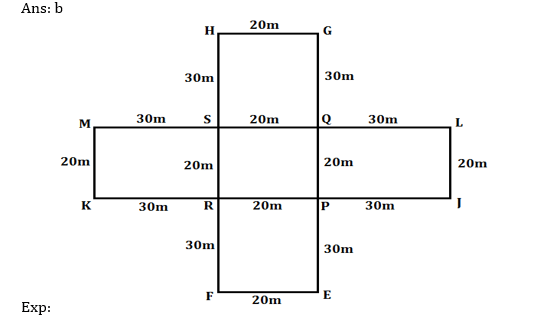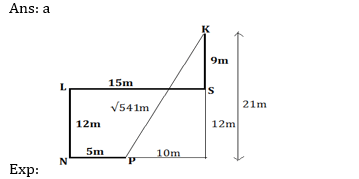# Direction Sense Based for IBPS, SBI, RBI, RRBs: Quiz 3

## Direction Sense Based for IBPS, SBI, RBI, RRBs: Quiz 3

Direction Sense Based plays a significant role in Reasoning Ability Section of banking exams such as IBPS, SBI and RBI PO and Clerk. You will get at least 3 to 5 questions from Direction Based in one of IBPS, SBI and RBI PO & clerk prelims and Mains exam. So, aspirants should focus on Direction Sense Based questions in detail. Here, we are providing you with the Direction Sense Based questions quiz with the detailed solution so that you can easily prepare for Direction Based questions. We are providing here all-important latest pattern-based questions and Previous Year Questions of Direction Based of various Government Exam like IBPS, SBI, and RBI PO and Clerk exam. This Direction Sense Based quiz we are providing is free. Attempt this Direction Based quiz to practice important questions with answers and solutions. And score better in IBPS, SBI and RBI PO and Clerk exam.

Direction Sense Based Quiz to improve your Reasoning Ability for SBI Po & SBI clerk exam IBPS PO Reasoning, IBPS Clerk Reasoning, IBPS RRB Reasoning, LIC AAO, LIC Assistant and another competitive exam.

Directions (1-2) Study the information carefully and answer the questions given below.

Zed and Jay are two cyclists who started from point A and P respectively at the speed of 10 km per hour.

Zed started moving towards the north direction and after travelling 2 km, he reached a point B which lies on the circumference of a circular track with radius 5km. From there he kept on moving along the circular track and after travelling half the circumference of the track, he reached point C where he found himself facing towards the east direction. He then stopped there to wait for Jay.

Jay started moving towards West direction and after travelling 5km he reached point Q. From Q he turned 45 degrees towards his right and travelled 10 km to meet Zed at point C.

Q1. What is the shortest distance between point Q and B?

(a) More than 12 km

(b) 8 km

(c) 10 km

(d) 12 km

(e) Cannot be determinedQ2. What is the shortest distance between point O and Q?Direction (3-4): Read the information carefully and answer the question:

A who faces North, started his journey from point X, he turns to his right and walks 8 km, after that A turns 90 degree anticlockwise direction and walks 6km to reach at point Y. From point Y, he turns to his left and walks 10km to reach at point Z.

Q3. What is the shortest distance between point X and Y?

(a) 8km

(b) 10m

(c) 10km

(d) None-of-these

(e) 12km1. Ans.(c)

Q4. What is the total distance covered by A from point X to point Z?

(a) 22km

(b) 24km

(c) 20km

(d) None-of-these

(e) 26km1. Ans.(b)

Direction (5-7): Study the following information carefully and answer the question given below:

EFGH is a rectangular ground such that E is in south of G. F is in south of H. Distance of EF is 20m and the longer side is 80m. E is in east of F.

JKLM is another rectangular ground such that J is in south of L and K is in south of M. Distance of JL is 20m and the longer side is 80m. J is in east of K.

EFGH rectangular intersects JKLM rectangular in such a way that EG intersects JK and LM at P and Q respectively. FH intersects JK and LM at R and S respectively (EP=QG similarly JP=RK).

1. A person enters in the ground from point P then start moving towards west direction. After covering 20m he turned towards his right then covered another 20m then he takes a left turn and after covering some distance from which of the following point he can exit from the ground?

(a) H.

(b) G.

(c) M.

(d) K.

(e) Q.1. What will be the total distance covered by a man who entered in the ground from point Q then reach to point S, from there reach point R and then exit from point K?

(a) 70m

(b) 80m

(c) 90m

(d) 100m

(e) None of these1. A person starts from point J towards north direction. After walks 20m, he turns towards left and then walks 30m. Now, he is standing in which direction with respect to H?

(a) East

(b) South-east

(c) North-east

(d) South

(e) North-westDirection (8-10): Study the following information carefully and answer the questions given below:

‘R # S’ means- Point S is 12m north of Point R.

‘R \$ S’ means- Point S is 9m south of Point R.

‘R & S’ means- Point S is 5m west of Point R.

‘R @ S’ means- Point S is 15m east of Point R.

1. If U # W & X \$ Y @ Z, then what is shortest distance and in which direction Point Z with respect to Point V, if Point V is 6m east of Point U?

(a) 5m, South-west

(b) 3m, North

(c) 11m, East

(d) 5m, North-east

(e) None of these9. If P & N # L @ S, K \$ S, then what is the shortest distance between Point K and Point P?10. If C & B \$ D @ A # E, F \$ C, F @ G & H, then Point E is in which direction with respect to Point H?

(a) North

(b) South-west

(c) North-east

(d) East

(e) None of theseDirection and Sense Quiz 3 PDF

#### Aatma Nirbhar Reasoning Topic Wise Online Test Series

Recommended PDF’s for:

#### Most important PDF’s for Bank, SSC, Railway and Other Government Exam : Download PDF Now

AATMA-NIRBHAR Series- Static GK/Awareness Practice Ebook PDF Get PDF here
The Banking Awareness 500 MCQs E-book| Bilingual (Hindi + English) Get PDF here
AATMA-NIRBHAR Series- Banking Awareness Practice Ebook PDF Get PDF here
Computer Awareness Capsule 2.O Get PDF here
AATMA-NIRBHAR Series Quantitative Aptitude Topic-Wise PDF Get PDF here
AATMA-NIRBHAR Series Reasoning Topic-Wise PDF Get PDF Here
Memory Based Puzzle E-book | 2016-19 Exams Covered Get PDF here
Caselet Data Interpretation 200 Questions Get PDF here
Puzzle & Seating Arrangement E-Book for BANK PO MAINS (Vol-1) Get PDF here
ARITHMETIC DATA INTERPRETATION 2.O E-book Get PDF here
3